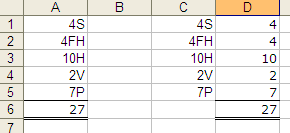Thanks:  0Likes:  0

# Thread: Remove Text, Keep Numbers in a cell

1. ##Remove Text, Keep Numbers in a cell

Hello,

I'm a bit of a newbie with Excel, but...

I have several cells with data that contain both letters and numbers (4H, 8V, 4FH, etc.) What I want to do is remove only the text characters from these cells, and add the remaining number values together among a series of cells with this data type.

If your answer involves using a macro or VB, please provide a link on how to use the formula. I've never used a macro or VB.

Thanks.Reply With Quote

2. ##Re: Remove Text, Keep Numbers in a cell

Are your numbers always one digit and at the start of the text as your examples imply. If yes...

If your data is in column A, put this in B1 and copy it down column B for each datum.

=LEFT(A1,1)Reply With Quote

3. ##Re: Remove Text, Keep Numbers in a cellOriginally Posted by leon6782Hello,

I'm a bit of a newbie with Excel, but...

I have several cells with data that contain both letters and numbers (4H, 8V, 4FH, etc.) What I want to do is remove only the text characters from these cells, and add the remaining number values together among a series of cells with this data type.

If your answer involves using a macro or VB, please provide a link on how to use the formula. I've never used a macro or VB.

Thanks.

Try http://www.asap-utilities.com/faq-questions-answers.phpReply With Quote

4. ##Re: Remove Text, Keep Numbers in a cellOriginally Posted by leon6782Hello,

I'm a bit of a newbie with Excel, but...

I have several cells with data that contain both letters and numbers (4H, 8V, 4FH, etc.) What I want to do is remove only the text characters from these cells, and add the remaining number values together among a series of cells with this data type.

If your answer involves using a macro or VB, please provide a link on how to use the formula. I've never used a macro or VB.

Thanks.

Try

http://www.asap-utilities.com/index.phpReply With Quote

5. ##Re: Remove Text, Keep Numbers in a cell

".) What I want to do is remove only the text characters from these cells, and add the remaining number values together among a series of cells with this data type."

Could you give us an example...

FWIW, and as an example of what can be done, if you have a range of strings in a1:a5 of the form nC (e.g. 1A, 2B etc), then:

=sumproduct(--(left(a1:a5,1)))

...will sum the numbers.Reply With Quote

6. ##Re: Remove Text, Keep Numbers in a cell

[QUOTE=
Could you give us an example...

QUOTE]

OK, here's a typical example. I have a series of cells with the following contents 4S , 4FH , 10H, 2V, 7P.

I looking for a formula that will remove the letters from these cells, then return the sum of just the numbers in cells...

In this case 4+4+10+2+7=27.

The LEFT function wont work because sometimes I need only the first digit, and sometimes it's the left 2 digits.

I know the CLEAN function removes all nonprintable characters and the TRIM function removes all spaces. Isn't there a function that removes all letters A thru Z?

How do these add ins work? If I buy an add-in program and use it in the spreadsheat I'm creating, will this same spreadsheet open correctly on someone else's computer who hasn't purchased the same add-in?

Any ideas would be greatly appreciated.

thanks.Reply With Quote

7. ##Re: Remove Text, Keep Numbers in a cell

If your data is in A1:A5, then maybe try something like this...

=SUM(IF(ISNUMBER(VALUE(LEFT(A1:A5,2))),VALUE(LEFT(A1:A5,2)),VALUE(LEFT(A1:A5,1))))

This is an array (CSE) formula. When you enter in this formula, use Ctrl+Shift+Enter. Excel will automatically put braces around the formula.

This works for up to two digit numbers that are at the start of your text.Reply With Quote

8. ##Re: Remove Text, Keep Numbers in a cell

Try this UDF:
Alt+F11
Insert Module
Copy/paste this:
Code:
```
Function SumIt(S As String) As Double
For Each V In Split(S)
SumIt = SumIt + Val(V)
Next
End Function```
In a cell write: =sumit(a1)Reply With Quote

9. ##Re: Remove Text, Keep Numbers in a cell

A helper column will also work with AlphaFrog's formula, without using an array formula (or needing a macro - though the last is neat):Formula in Cell A6 (Alpha Frog's Formula, array entered):
=SUM(IF(ISNUMBER(VALUE(LEFT(A1:A5,2))),VALUE(LEFT(A1:A5,2)),VALUE(LEFT(A1:A5,1))))

Formula in Cell D1 (copied down to D5):
=IF(ISNUMBER(VALUE(LEFT(C1,2))),VALUE(LEFT(C1,2)),VALUE(LEFT(C1,1)))
Formula in Cell D6:
=Sum(D1:D5)Reply With Quote

10. ##Re: Remove Text, Keep Numbers in a cellOriginally Posted by AlphaFrogIf your data is in A1:A5, then maybe try something like this...

=SUM(IF(ISNUMBER(VALUE(LEFT(A1:A5,2))),VALUE(LEFT(A1:A5,2)),VALUE(LEFT(A1:A5,1))))

This is an array (CSE) formula. When you enter in this formula, use Ctrl+Shift+Enter. Excel will automatically put braces around the formula.

This works for up to two digit numbers that are at the start of your text.

AlphaFrog, thanks for your help. Your formula is giving me a #VALUE result. I forgot to metion that I also have single digit values, two digit values, single letter values, and two letter values that will sometimes appear in my cells used in the calculation. So I will also have values like 8, 10, V, FH.

Could this be messing with your formula? If so, is there a way your formula could be modified to accomodate these other types of values?

If you can think of anything, please let me know.

Thanks.Reply With Quote

## User Tag List

####Posting Permissions

• You may not post new threads
• You may not post replies
• You may not post attachments
• You may not edit your posts
•